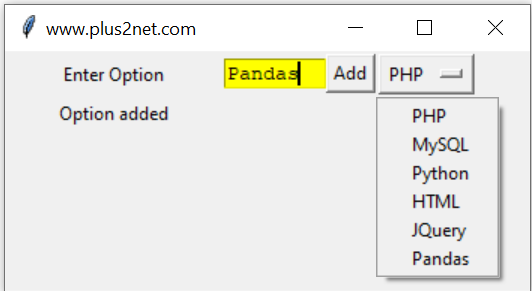## Components in our GUI window

We will have one text box , one Button and one OptionMenu. User can enter data in the textbox and on click of Add button the entered data will be added as option in our OptionMenu.
Component ( Text ) FunctionAction performed by the Function
l1 ( Enter Option ) -Display Message only before the OptionMenu
om1 --The OptionMenu to which the entered text will be added as Option
l2 ( Output ) --Display messages if Option is added or not added ( already available )
Here is the code to add the component to our GUI window.
``````import tkinter as tk
my_w = tk.Tk()
my_w.geometry("350x150")  # Size of the window

my_list = ["PHP","MySQL","Python","HTML","JQuery"]
options = tk.StringVar(my_w)
options.set(my_list) # default value

l1 = tk.Label(my_w,  text='Enter Option', width=10 )  # added one Label
l1.grid(row=2,column=1)

t1 = tk.Text(my_w,  height=1, width=8,bg='yellow') # added one text box
t1.grid(row=2,column=2)

b1.grid(row=2,column=4)

om1.grid(row=2,column=5)

my_str = tk.StringVar()

l2 = tk.Label(my_w,  text='Output',textvariable=my_str, width=20 )  # added one Label
l2.grid(row=3,column=1) ``````
In above code we have used on click event of our Button b1 to execute the function my_add()

This function is triggered when Button b1 is clicked. First we will create one list by using the available options in our OptionMenu om1. We used one for loop to add one by one option to our list ( items ) by using its index position.
``````def my_add():
items = []
for index in range(last+1):
We will read the text entered by user. ( you can add any minimum length requirement here )
``opt=t1.get("1.0",'end-1c') # read value entered by user``
By using one if else condition check we can find out if the user entered data is already available as an option. If it is not there then we will add to the OptionMenu , otherwise we will give message saying already available.
``````if opt in items:
else:
options.set(my_list) # default value set
Full code is here
``````import tkinter as tk
my_w = tk.Tk()
my_w.geometry("350x150")  # Size of the window

my_list = ["PHP","MySQL","Python","HTML","JQuery"]
options = tk.StringVar(my_w)
options.set(my_list) # default value

l1 = tk.Label(my_w,  text='Enter Option', width=10 )  # added one Label
l1.grid(row=2,column=1)

t1 = tk.Text(my_w,  height=1, width=8,bg='yellow') # added one text box
t1.grid(row=2,column=2)

b1.grid(row=2,column=4)

om1.grid(row=2,column=5)

my_str = tk.StringVar()

l2 = tk.Label(my_w,  text='Output',textvariable=my_str, width=20 )  # added one Label
l2.grid(row=3,column=1)

items = []
for index in range(last+1):
# items is the list with all available options of OptionMenu om1
#print(items)
opt=t1.get("1.0",'end-1c') # read value entered by user
if opt in items:
else:
options.set(my_list) # default value set
my_w.mainloop()``````

Subscribe to our YouTube Channel here

## Subscribe

* indicates required
Subscribe to plus2netplus2net.com